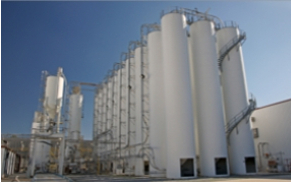TEC Engineering Web

# CAN YOU ANSWER THE PUZZLER?

## Lab Rat### Question

I was working in a chemistry lab and created a mixture whose weight was 90% water and 10% carbon. The total weight of the mixture was 20 pounds.

After creating the mixture, I left for 2 days. When I returned, the weight of water in the mixture was reduced from 90% to 50%.

How much does the mixture weigh now?

4 Pounds

Explanation:

90% of water = 18 pounds
10% of carbon = 2 pounds

Now, the 10% of carbon is contributing 50% weight = 2 pounds
Therefore, the weight of water is also contributing 50% weight = 2 pounds
Sum = 4 pounds

TEC Engineering office
is located in

[+] Change
[-]Close

TEC Engineering linesheet.

View our